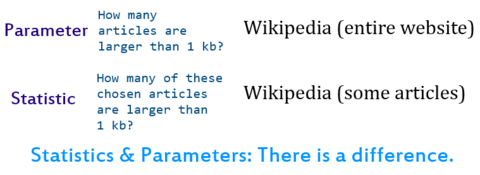# Statistic

For other uses, see Statistics (disambiguation).

A statistic (singular) or sample statistic is a single measure of some attribute of a sample (e.g., its arithmetic mean value). It is calculated by applying a function (statistical algorithm) to the values of the items of the sample, which are known together as a set of data.

More formally, statistical theory defines a statistic as a function of a sample where the function itself is independent of the sample's distribution; that is, the function can be stated before realization of the data. The term statistic is used both for the function and for the value of the function on a given sample.

A statistic is distinct from a statistical parameter, which is not computable because often the population is much too large to examine and measure all its items. However, a statistic, when used to estimate a population parameter, is called an estimator. For instance, the sample mean is a statistic that estimates the population mean, which is a parameter.

When a statistic (a function) is being used for a specific purpose, it may be referred to by a name indicating its purpose: in descriptive statistics, a descriptive statistic is used to describe the data; in estimation theory, an estimator is used to estimate a parameter of the distribution (population); in statistical hypothesis testing, a test statistic is used to test a hypothesis. However, a single statistic can be used for multiple purposes – for example the sample mean can be used to describe a data set, to estimate the population mean, or to test a hypothesis.

## Examples

In calculating the arithmetic mean of a sample, for example, the algorithm works by summing all the data values observed in the sample and then dividing this sum by the number of data items. This single measure, the mean of the sample, is called a statistic; its value is frequently used as an estimate of the mean value of all items comprising the population from which the sample is drawn. The population mean is also a single measure; however, it is not called a statistic, because it is not obtained from a sample; instead it is called a population parameter, because it is obtained from the whole population.

Other examples of statistics include

## Properties

### Observability

A statistic is an observable random variable, which differentiates it both from a parameter that is a generally unobservable quantity describing a property of a statistical population, and from an unobservable random variable, such as the difference between an observed measurement and a population average. A parameter can only be computed exactly if the entire population can be observed without error; for instance, in a perfect census or for a population of standardized test takers.

Statisticians often contemplate a parameterized family of probability distributions, any member of which could be the distribution of some measurable aspect of each member of a population, from which a sample is drawn randomly. For example, the parameter may be the average height of 25-year-old men in North America. The height of the members of a sample of 100 such men are measured; the average of those 100 numbers is a statistic. The average of the heights of all members of the population is not a statistic unless that has somehow also been ascertained (such as by measuring every member of the population). The average height that would be calculated using the all of the individual heights of all 25-year-old North American men is a parameter, and not a statistic.

### Statistical properties

Important potential properties of statistics include completeness, consistency, sufficiency, unbiasedness, minimum mean square error, low variance, robustness, and computational convenience.

### Information of a statistic

Information of a statistic on model parameters can be defined in several ways. The most common is the Fisher information, which is defined on the statistic model induced by the statistic. Kullback information measure can also be used.

## Common misconceptionsA diagram that deals with the difference between statistic and parameter.Look up statistic in Wiktionary, the free dictionary.